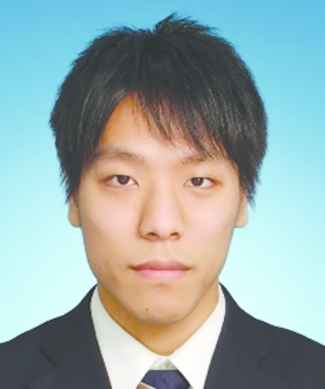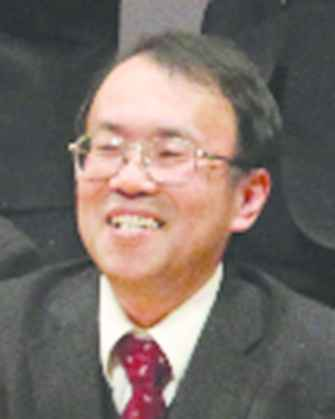# Journal of Robotics, Networking and Artificial Life

Volume 5, Issue 4, March 2019, Pages 257 - 260

# Design of a Data-Driven Controller with Evaluating Controller Performance

Authors
Takuya Kinoshita*, Toru Yamamoto
Graduate School of Engineering, Hiroshima University, Higashihiroshima, Hiroshima, Japan
*Corresponding author. Email: kinoshita-takuya@hiroshima-u.ac.jp
Corresponding Author
Takuya Kinoshita
Received 10 November 2018, Accepted 21 November 2018, Available Online 30 March 2019.
DOI
10.2991/jrnal.k.190220.011How to use a DOI?
Keywords
PID controller; controller assessment; data-driven controller; least squares method
Abstract

Data-driven controller systems have been proposed to achieve the desired control performance without using any system identifications. The effectiveness of these control schemes has been shown through experimental results. For time-variant and nonlinear systems, it is important to evaluate the controller performance and redesign controller when the performance is poor. According to the proposed scheme, the controller performance calculator and controller design are integrated using only input and output data.

Open Access

## 1. INTRODUCTION

In most industries, it is very important to get desired control performance. Data-driven control schemes  have been proposed to achieve the aforementioned desired control performance without using any system identifications. For time-variant systems, performance-driven controller  has been proposed to improve the steady state control performance only when the performance is poor. However, performance-driven controller cannot evaluate the transient state because this scheme focuses on the variance of steady state error.

In this paper, the scheme of evaluating controller performance without using any system identifications is proposed. According to the proposed scheme, the control performance is evaluated system output error including transient state. Furthermore, the controller performance and controller parameters are simultaneously calculated using only input and output data.

## 2. OUTLINE FIGURE OF THE PROPOSED SCHEME

The schematic figure of the proposed control system is shown in Figure 1. C(z−1), G(z−1), Gm(z−1), and Ĝ(z−1) are the controller, controlled system, reference model and estimated system model, respectively.

The purpose of the proposed control system is to achieve the desired control performance by minimizing the following criterion Jr [Equations (1) and (2)]:

Jr=12ϕr(t)2
ϕr(t)=y(t)yr(t),
where y(t) and yr(t) are the control output and reference output, respectively.

Another purpose of the proposed scheme is to design the estimated system model Ĝ(z−1) by minimizing the following Equations (3) and (4):

Jr=12ϕ^(t)2
ϕ^(t)=y(t)y^(t),
where ŷ(t) is the estimated output. In the proposed scheme, Ĝ(z−1) can be introduced by using the controller parameters and reference model parameters without any system identifications. Therefore, controller and estimated system model can be designed simultaneously.

In addition, users set a desired reference model expressed by the following Equations (5) and (6):

yr(t)=Gm(z1)r(t)
Gm(z1):=z(1+d)P(1)P(z1)
where r(t) is the reference signal and P(z−1) is user-specified polynomial. P(z−1) is designed based on the reference design  as follows [Equations (7) and (8)]:
P(z1):=1+p1z1+p2z1
{p1=2exp(ρ2μ)cos(4μ12μρ)p2=exp(ρμ)ρ:=Tsσμ:=0.25(1δ)+0.51δ
where σ is a parameter related to the rise-time and δ is a parameter related to the damping oscillation. User set them arbitrarily. σ denotes the time when output reaches about 60% of the step reference value. Moreover, δ is set between 0 ≤ δ ≤ 2.0 desirably. In particular, δ = 0 indicates the response of Butterworth model and δ = 1.0 indicates the response of binominal model.

## 3. RELATIONSHIP BETWEEN CONTROLLER AND ESTIMATED SYSTEM MODEL

The following Equation (9) can be obtained by introducing the optimized controller C*(z−1) which achieves ϕr (t) = 0:

G(z1)C*(z1)1+G(z1)C*(z1)=Gm(z1).

The controlled system G(z−1) is expressed as follows by using the aforementioned Equation (10):

G(z1)=Gm(z1)C*(z1){1Gm(z1)}.

Here, the following estimated system model Ĝ(z−1) is defined using a controller C(z−1) instead of C*(z−1) because it is difficult to obtain the optimized controller C*(z−1) [Equation (11)]:

G^(z1)=Gm(z1)C(z1){1Gm(z1)}.

Note that the estimated controlled system Ĝ(z−1) is expressed using a controller C(z−1) and reference model Gm(z−1) without any system identifications.

Ĝ(z−1) equals to G(z−1) when the optimized controller C*(z−1) is obtained. It mentions that ϕ^(t)=0 because of Ĝ(z−1) = G(z−1). Therefore, the optimized controller C*(z−1) achieves ϕr (t) = 0 and ϕ^(t)=0 simultaneously.

## 3.1. Evaluation of the Controller Performance

The optimized controller C*(z−1) makes ϕ^(t) equals to zero. In contrast, ϕ^(t) becomes large when the performance of the controller C(z−1) is poor. Hence, this paper considers the performance of the controller C(z−1) based on ϕ^(t) .

## 3.2. Tuning Scheme of the PID Gains

In this paper, the controller is utilized as following I-PD controller [Equations (12) and (13)]:

Δu(t)=KIe(t)KPΔy(t)KDΔ2y(t)
e(t):=r(t)y(t),
where KP, KI, and KD are the proportional gain, integral gain and derivative gain, respectively.

The estimated system model Ĝ(z−1) is expressed by using I-PD controller is as follows [Equation (14)]:

G^(z1)=Gm(z1)KIΔC(z1)Gm(z1),
where C(z−1) is as follows [Equation (15)]:
C(z1)=KPΔ+KI+KDΔ2Δ.

The estimated output is calculated as follows [Equation (16)]:

y^(t)=Gm(z1)KIΔC(z1)Gm(z1)u(t),
where u(t) is control input. To apply the least squares method, ϕ^(t)=0 is considered and the following Equation (17) is derived by using Equations (2) and (18):
Gm(z1)u(t)={KIΔKPΔ+KI+KDΔ2ΔGm(z1)}y(t)
ΔGm(z1)u(t)=KI{1Gm(z1)}y(t)(KPΔ+KDΔ2)Gm(z1)y(t).

Therefore, the PID gains are calculated by using the following least squares method [Equations (19) and (20)]:

θ=(ΦTΦ)1ΦTν,
{ψ(t)=[ΔGm(z1)y(t){1Gm(z1)}y(t)Δ2Gm(z1)y(t)]TΦ=[ψ(1),ψ(2),,ψ(N)]Tν=[ΔGm(z1)u(1),,ΔGm(z1)u(N)]Tθ=[KP,KI,KD]T

## 4. NUMERICAL EXAMPLE

The controlled system G(s) is given as follows [Equation (21)]:

{G(s)=11+10s(t<100)G(s)=21+20s(t100).

The parameters of the reference model Gm (z−1) are set as follows [Equation (22)]:

σ=3.0,δ=0.

Finally, the initial PID gains are set as follows [Equation (23)]:

KP=1.0,KI=1.0,KD=1.0.

In this section, the following three simulations are shown.

1. A)

Figure 2: Control result by using initial PID gains of Equation (23).

2. B)

Figure 3: Control result of the proposed scheme without controller retuning.

3. C)

Figure 5: Control result of the proposed scheme with controller retuning.

## 4.1. Control Results

Figure 2 shows the control result by using initial PID gains of Equation (23). The control performance is poor because control output y(t) does not track to reference output yr(t).

Figure 3 shows the control result of the proposed scheme. The following PID gains [Equation (24)] were calculated applying Equation (19) by using the data between t = 0 and 100 [step] in Figure 2:

KP=6.74,KI=2.49,KD=0.0.

In Figure 3, the control performance is good even though the system G(z−1) is changed at t = 100 [step]. It mentions that it is difficult to detect the system parameters are changed. The reason for the abovementioned, the feedback controller C(z−1) has an integrator, and it can reduce the steady state error. On the other hand, Figure 4 shows the trajectories of the estimated output ŷ(t). It easier to detect the system parameters are changed than Figure 3.

Finally, Figure 5 shows the control result with controller retuning at 120 [step]. Figure 6 shows the estimated output ŷ(t), and Figure 7 shows the trajectories of the PID gains corresponding to Figure 5. In Figure 7, each PID gains are slightly adjusted, however, the estimated output ŷ(t) of Figure 6 is significantly improved. Therefore, the proposed scheme can diagnose the system parameters are changed strictly.

## 5. CONCLUSION

This paper has proposed the design of data-driven control system with evaluating controller performance. The features of the proposed scheme are as follows:

• Controller and estimated system model can be designed simultaneously.

• Controller performance is evaluated by the error between output y(t) and estimated output ŷ(t) without using any system identifications.

The proposed scheme has been verified by numerical examples.

## Authors Introduction

Dr. Takuya KinoshitaHe received his B. Eng., M. Eng., and D. Eng. from Hiroshima University in Japan in 2013, 2015, and 2017, respectively. He was postdoctoral fellow of JSPS (Japan Society for the Promotion of Science) in 2017. He is currently an Assistant Professor with the Department of System Cybernetics, Graduate School of Engineering, Hiroshima University, Japan. His research interests are performance driven control.

Prof. Toru YamamotoHe received the B. Eng. and M. Eng. degrees from the University of Tokushima, Tokushima, Japan, in 1984 and 1987, respectively, and the D. Eng. degree from Osaka University, Osaka, Japan, in 1994. He is currently a Professor with the Department of System Cybernetics, Graduate School of Engineering, Hiroshima University, Japan. He was a Visiting Researcher with the Department of Mathematical Engineering and Information Physics, University of Tokyo, Tokyo, Japan, in 1991. He was an Overseas Research Fellow of the Japan Society for Promotion of Science with the University of Alberta for six months in 2006. His current research interests are in the area of data-driven control, and process control. Dr. Yamamoto was the recipient of the Commendation for Science and Technology by the Minister of Education, Culture, Sports and Technology in 2009.